# How to Drop Columns with NaN Values in Pandas DataFrame?

• Last Updated : 24 Oct, 2020

Nan(Not a number) is a floating-point value which can’t be converted into other data type expect to float. In data analysis, Nan is the unnecessary value which must be removed in order to analyze the data set properly. In this article, we will discuss how to remove/drop columns having Nan values in the pandas Dataframe. We have a function known as Pandas.DataFrame.dropna() to drop columns having Nan values.

Syntax: DataFrame.dropna(axis=0, how=’any’, thresh=None, subset=None, inplace=False)

Example 1: Dropping all Columns with any NaN/NaT Values.

## Python3

 `# Importing libraries``import` `pandas as pd``import` `numpy as np`` ` `# Creating a dictionary``dit ``=` `{``'August'``: [pd.NaT, ``25``, ``34``, np.nan, ``1.1``, ``10``],``       ``'September'``: [``4.8``, pd.NaT, ``68``, ``9.25``, np.nan, ``0.9``],``       ``'October'``: [``78``, ``5.8``, ``8.52``, ``12``, ``1.6``, ``11``], }`` ` `# Converting it to data frame``df ``=` `pd.DataFrame(data``=``dit)`` ` `# DataFrame``df`

Output: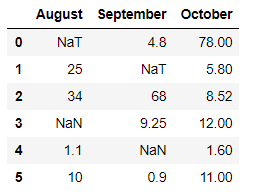## Python3

 `# Dropping the columns having NaN/NaT values``df ``=` `df.dropna(axis``=``1``)`` ` `df`

Output: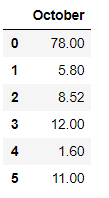In the above example, we drop the columns ‘August’ and ‘September’ as they hold Nan and NaT values.

Example 2: Dropping all Columns with any NaN/NaT Values and then reset the indices using the df.reset_index() function.

## Python3

 `# Importing libraries``import` `pandas as pd``import` `numpy as np`` ` `# Initializing the nested list with Data set``player_list ``=` `[[``'M.S.Dhoni'``, ``36``, ``75``, ``5428000``], ``               ``[np.nan, ``36``, ``74``, np.nan],``               ``[``'V.Kholi'``, ``31``, ``70``, ``8428000``],``               ``[``'S.Smith'``, ``34``, ``80``, ``4428000``], ``               ``[pd.NaT, ``39``, ``100``, np.nan],``               ``[np.nan, ``33``, ``90.5``, ``7028000``],``               ``[``'K.Peterson'``, ``42``, ``85``, pd.NaT]]`` ` `# creating a pandas dataframe``df ``=` `pd.DataFrame(player_list, columns``=``[``'Name'``, ``'Age'``, ``                                        ``'Weight'``, ``'Salary'``])`` ` `df`

Output: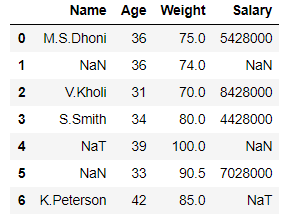## Python3

 `# Dropping the columns having NaN/NaT values``df ``=` `df.dropna(axis``=``1``)`` ` `# Resetting the indices using df.reset_index()``df ``=` `df.reset_index(drop``=``True``)`` ` `df`

Output: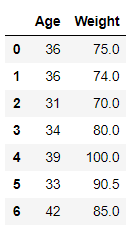In the above example, we drop the columns ‘Name’ and ‘Salary’ and then reset the indices.

Example 3:

## Python3

 `# Importing libraries``import` `pandas as pd``import` `numpy as np`` ` `# creating and initializing a nested list``age_list ``=` `[[np.nan, ``1952``, ``8425333``, np.nan, ``28.35``], ``            ``[``'Australia'``, ``1957``, ``9712569``, ``'Oceania'``, ``24.26``],``            ``[``'Brazil'``, ``1962``, ``76039390``, np.nan, ``30.24``],``            ``[pd.NaT, ``1957``, ``637408000``, ``'Asia'``, ``28.32``], ``            ``[``'France'``, ``1957``, ``44310863``, pd.NaT, ``25.21``],``            ``[``'India'``, ``1952``, ``3.72e``+``08``, pd.NaT, ``27.36``], ``            ``[``'United States'``, ``1957``, ``171984000``, ``'Americas'``, ``28.98``]]`` ` `# creating a pandas dataframe``df ``=` `pd.DataFrame(age_list, columns``=``[``                  ``'Country'``, ``'Year'``, ``'Population'``, ``'Continent'``, ``'lifeExp'``])`` ` `df`

Output: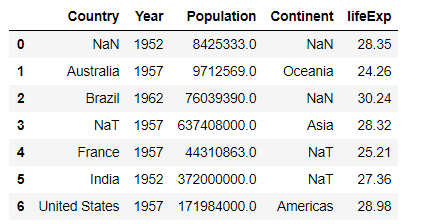## Python3

 `# Dropping the columns having NaN/NaT values``df ``=` `df.dropna(axis``=``1``)`` ` `# Resetting the indices using df.reset_index()``df ``=` `df.reset_index(drop``=``True``)`` ` `df`

Output: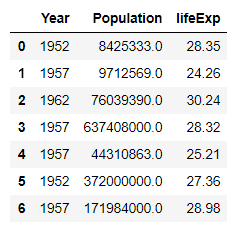In the above example, we drop the columns ‘Country’ and ‘Continent’ as they hold Nan and NaT values.

Example 4: Dropping all Columns with any NaN/NaT Values under a certain label index using ‘subset‘ attribute.

## Python3

 `# Importing libraries ``import` `pandas as pd ``import` `numpy as np `` ` `# Creating a dictionary ``dit ``=` `{``'August'``: [``10``, np.nan, ``34``, ``4.85``, ``71.2``, ``1.1``], ``       ``'September'``: [np.nan, ``54``, ``68``, ``9.25``, pd.NaT, ``0.9``], ``        ``'October'``: [np.nan, ``5.8``, ``8.52``, np.nan, ``1.6``, ``11``],``       ``'November'``: [pd.NaT, ``5.8``, ``50``, ``8.9``, ``77``, pd.NaT] }`` ` `# Converting it to data frame``df ``=` `pd.DataFrame(data``=``dit)`` ` `# data frame``df`

Output: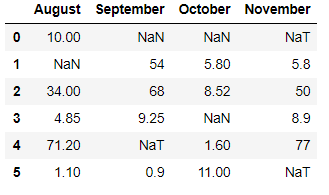## Python3

 `# Dropping the columns having NaN/NaT values``# under certain label index using  'subset' attribute``df ``=` `df.dropna(subset``=``[``3``], axis``=``1``)`` ` `# Resetting the indices using df.reset_index()``df ``=` `df.reset_index(drop``=``True``)`` ` `df`

Output: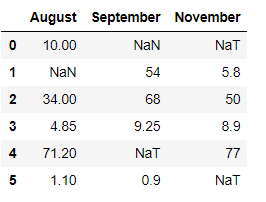In the above example, we drop the column having index 3 i.e ‘October’ using subset attribute.

My Personal Notes arrow_drop_up